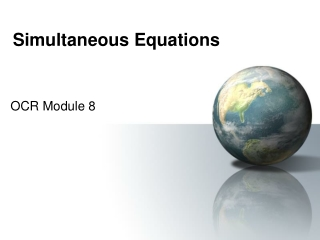DownloadDownload PresentationSimultaneous Equations

# Simultaneous Equations

Télécharger la présentation## Simultaneous Equations

- - - - - - - - - - - - - - - - - - - - - - - - - - - E N D - - - - - - - - - - - - - - - - - - - - - - - - - - -
##### Presentation Transcript

1. Simultaneous Equations OCR Module 8

2. What are they? • Simply 2 equations • With 2 unknowns • Usually x and y • To SOLVE the equations means we find values of x and y that • Satisfy BOTH equations [work in] • At same time [simultaneously]

3. Elimination Method We have the same number of y’s in each 2x – y = 1 A + If we ADD the equations, the y’s disappear B 3x + y = 9 = 10 5x Divide both sides by 5 = 2 x 2 x 2 – y = 1 Substitute x = 2 in equation A 4 – y = 1 Answer x = 2, y = 3 y = 3

4. Elimination Method We have the same number of y’s in each 5x + y = 17 A - B 3x + y = 11 If we SUBTRACT the equations, the y’s disappear = 6 2x Divide both sides by 2 = 3 x 5 x 3 + y = 17 Substitute x = 3 in equation A 15 + y = 17 Answer x = 3, y = 2 y = 2

5. Elimination Method We have the same number of x’s in each 2x + 3y = 9 A - B 2x + y = 7 If we SUBTRACT the equations, the x’s disappear = 2 2y Divide both sides by 2 = 1 y 2x + 3 = 9 Substitute y = 1 in equation A 2x = 6 Answer x = 3, y = 1 x = 3

6. Elimination Method We have the same number of y’s in each 4x - 3y = 14 A + B 2x + 3y = 16 If we ADD the equations, the y’s disappear 6x = 30 Divide both sides by 6 x = 5 20 – 3y = 14 Substitute x = 5 in equation A 3y = 6 Answer x = 5, y = 2 y = 2

7. Basic steps • Look at equations • Same number of x’s or y’s? • If the sign is different, ADD the equations otherwise subtract tem • Then have ONE equation • Solve this • Substitute answer to get the other • CHECK by substitution of BOTH answers

8. What if NOT same number of x’s or y’s? A 3x + y = 10 If we multiply A by 2 we get 2y in each B 5x + 2y = 17 A - 6x + 2y = 20 B 5x + 2y = 17 x = 3 In B 5 x 3 + 2y = 17 Answer x = 3, y = 1 15 + 2y = 17 y = 1

9. What if NOT same number of x’s or y’s? A 4x - 2y = 8 If we multiply A by 3 we get 6y in each B 3x + 6y = 21 A 12x - 6y = 24 + B 3x + 6y = 21 15x = 45 x = 3 In B 3 x 3 + 6y = 21 Answer x = 3, y = 2 6y = 12 y = 2

10. …if multiplying 1 equation doesn’t help? A 3x + 7y = 26 Multiply A by 5 & B by 3, we get 15x in each B 5x + 2y = 24 A 15x + 35y = 130 - B 15x + 6y = 72 Could multiply A by 2 & B by 7 to get 14y in each = 58 29y y = 2 In B 5x + 2 x 2 = 24 Answer x = 4, y = 2 5x = 20 x = 4

11. …if multiplying 1 equation doesn’t help? A 3x - 2y = 7 Multiply A by 3 & B by 2, we get +6y & -6y B 5x + 3y = 37 A 9x – 6y = 21 + B 10x + 6y = 74 Could multiply A by 5 & B by 3 to get 15x in each = 95 19x x = 5 In B 5 x 5 + 3y = 37 Answer x = 5, y = 4 3y = 12 y = 4